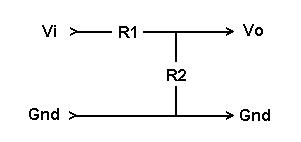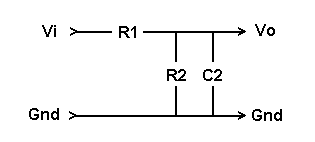Daqarta
Data AcQuisition And Real-Time Analysis
Scope - Spectrum - Spectrogram - Signal Generator
Software for WindowsThe following is from the Daqarta Help system:

# Sound Card Impedance Measurement

## Introduction:

This topic discusses how to measure an impedance that can be "lumped" into a single resistance and capacitance. In particular, it deals with measuring the input and output impedances of a sound card.

## Sound Card Input Impedance:

The input impedance of a device is typically regarded as a resistor and capacitor in parallel with each other from the input terminal to ground. To measure these values, the device is driven through a resistor in series with the input, from a source with a known output impedance.

To simplify calculations, we will assume that the source output impedance is zero. This is a reasonable assumption as long as it is well under 1% of the input impedance to be measured, which is ususally the case with sound cards: Typical output impedances are under 100 ohms, and input impedances are over 10000 ohms. This means that any errors due to the assumption will be under 1%.

We'll also completely ignore the large DC blocking capacitors on both the input and output, which cut off frequencies below (typically) a few hertz. We'll be working at higher frequencies where their effects will be insignificant.

To understand the basic method, begin by assuming that the input impedance is a pure resistance from the input to ground. (There's also an effective input capacitance in parallel with that, which we'll consider in a later step.) Thus, if a resistor is put in series with the input, the result is a simple voltage divider:Here R1 is the series resistor, and R2 is the effective resistance of the input stage. Vi is the input to the divider from the output of the sound card. Vo is the output from the divider to the effective input of the sound card.

Make an initial measurement with R1 replaced by a direct connection. To do that, load the Daqarta Generator with the Default.GEN setup, which is a 440 Hz Sine output that has only the Left output active. That actually sends the same (Left) signal to both outputs, so toggle the Right output on. Since the Right channel as no active Stream, there will be no Right output signal.

From the Input control dialog (CTRL+I) select Line Input, and set the Right Input Level to minimum (typically -191).

Now adjust Generator Volume (F9 key) and the Left Input Level for a large but undistorted waveform on the Left Input channel. Note that many sound card inputs distort at much less than full scale... you'll see the top and bottom of the sine wave flatten when the input is overdriven, or you can switch to Spectrum mode and see a sudden onset of harmonic distortion components.

When you have a large non-distorting wave, open the Daqarta Voltmeter (ALT+V) and select the LI channel and RMS mode. For these impedance measurements it doesn't matter if your card is calibrated to read absolute voltage yet, since only ratios of readings are involved.

Record the reading and call it Vi. This is the same voltage that will be at the input of the voltage divider once R1 is in place.

Insert R1 and take another reading, and call it Vo. Ideally you should use an R1 value in the general range of the expected input resistance R2. 100K ohms (100,000 ohms) is a good value for R1, although you can use anything from (say) 10K to 500K here. The exact value isn't critical as long as you know what it is.

Refer to the Voltage Divider topic, and compute R2 from:

R2 = Vo * R1 / (Vi - Vo)

R2 is the resistive part of the sound card input impedance. The capacitive part C2 will be assumed to be in parallel with R2, as shown:NOTE: The measured value for C2 will include the capacitance of any cable between R1 and the sound card input. This may be much greater than the actual sound card input capacitance. (The capacitance of the cable from the sound card output has a negligible effect on the measurement.) Cable capacitance is roughly proportional to cable length, and also varies with the type of cable. Make sure your measurement reflects the way the sound card will actually be used, with the same input cable if possible.

Ignoring R2 for the moment, notice that R1 and C2 form a simple low-pass filter. Adding R2 back is (for purposes of filter frequency calculations) the same as putting it in parallel with R1. (The fact that one end is grounded means it acts like a second filter input resistor from a zero-volt source. But the circuit analysis involves setting all voltage sources to zero, so the original input resistor would be effectively grounded as well.)

You can measure the cutoff frequency of this filter using any of the standard frequency response methods. A swept response is cleanest; set the sweep from (say) 10 Hz to 22 kHz over a 10-second Sweep Length. Be sure to set the Spectrum Window to Flat-Top. Set the Spectrum Averager to Peak mode, and average 1000 frames.

Set the solid cursor at a low frequency like 100-400 Hz. Set the little colored cursor buttons to match the Left In trace color (yellow default), and make sure the Delta readout mode is active (default).

Now drag (or use SHIFT plus the left/right arrow keys) to move the dotted cursor until the Delta readout shows -3 dB relative to the solid cursor. The dotted cursor frequency readout now shows the cutoff frequency of the filter, which we'll call Fc.

To find the effective capacitor value, first compute the equivalent resistance Rp of R1 and R2 in parallel:

Rp = (R1 * R2) / (R1 + R2)

Then use this to find C2 via:

C2 = 1 / (2 * pi * Rp * Fc)

## Typical Sound Card Input Impedance Measurements:

With a direct connection instead of R1, the Vi RMS voltage was 335.4 mV (uncalibrated) for a 440 Hz sine with levels adjusted to just below clipping. With 100K for R1, the Vo reading was 134.6 mV.

R2 = Vo * R1 / (Vi - Vo)

R2 = 134.6 * 100K / (335.4 - 134.6)

R2 = 134.6 * 100K / 200.8

R2 = 0.6703 * 100K

R2 = 67.03K

With a 10 inch cable between R1 and the sound card input, the frequency sweep showed a -3.025 dB drop at 12375 Hz relative to a solid cursor reference at 421.875 Hz.

The parallel combination of R1 and R2 was:

Rp = (100K * 67.03K) / (100K + 67.03K)

Rp = 6703K / 167.03K

Rp = 40.13K

The input capacitance C2 was found via:

C2 = 1 / (2 * pi * pi * Fc)

C2 = 1 / (2 * 3.1416 * 40130 * 12375)

C2 = 1 / 3.12*10^9

C2 = 320*10^-12

C2 = 320 pF

With a 6 foot cable between R1 and the sound card input, the cutoff frequency was 6328 Hz, giving a C2 value of 627 pF.

With a 12 foot cable the cutoff was 3703 Hz, giving a C2 value of 1071 pF.

## Sound Card Output Impedance:

As mentioned under Input Impedance, we will ignore DC blocking capacitors. We will assume that the output impedance of the sound card is a pure resistance with no capacitive component at the audio frequencies of interest.

This is a good assumption because the resistance value is typically very low; the low-pass filter formed by any capacitance to ground will have a cutoff frequency well beyond the audio range.

The measurement technique uses the same voltage divider method:Here, R1 is the effective output resistance and R2 is a test load resistor. Vi is the input to the divider from the ideal output of the sound card. Vo is the actual output from sound card, which is the output of the divider to the effective input of the sound card.

The initial measurement is made without R2, meaning it is exactly the same direct connection from output to input as the initial measurement for input impedance discussed above. Set everything the same: 440 Hz sine, with Input and Output levels adjusted for no clipping. Record the Voltmeter RMS value as Vi.

Now add R2 from the output to ground. This should be a value in the general range of the expected output resistance; 100 ohms is a good value to use, but again the exact value is not important as long as you know what it is.

Record the new voltage as Vo and solve for R1 with:

R1 = R2 * (Vi - Vo) / Vo

## Typical Sound Card Output Impedance Measurement:

Without R2, the Vi RMS voltage was 335.2 mV (uncalibrated) for a 440 Hz sine with levels adjusted to just below clipping. With 100 ohms for R2, the Vo reading was 227.2 mV.

R1 = R2 * (Vi - Vo) / Vo

R1 = 100 * (335.2 - 227.2) / 227.2

R1 = 100 * 0.475

R1 = 47.5 ohmsGO: